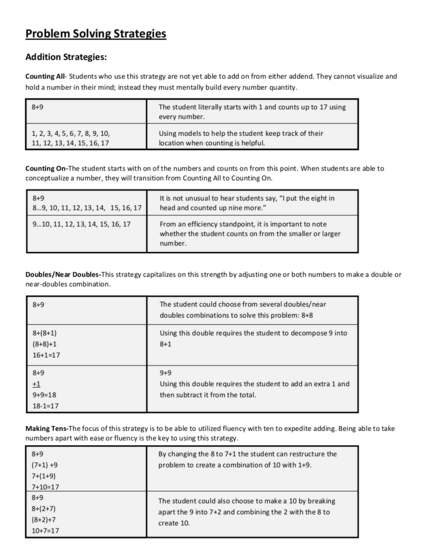# Problem Solving StrategiesContributed by:This pdf includes following topics:-
Counting On
Making Landmark or Friendly Numbers
Compensation
Examples
1. Problem Solving Strategies
Counting All- Students who use this strategy are not yet able to add on from either addend. They cannot visualize and
hold a number in their mind; instead they must mentally build every number quantity.
8+9 The student literally starts with 1 and counts up to 17 using
every number.
1, 2, 3, 4, 5, 6, 7, 8, 9, 10, Using models to help the student keep track of their
11, 12, 13, 14, 15, 16, 17 location when counting is helpful.
Counting On-The student starts with on of the numbers and counts on from this point. When students are able to
conceptualize a number, they will transition from Counting All to Counting On.
8+9 It is not unusual to hear students say, “I put the eight in
8…9, 10, 11, 12, 13, 14, 15, 16, 17 head and counted up nine more.”
9…10, 11, 12, 13, 14, 15, 16, 17 From an efficiency standpoint, it is important to note
whether the student counts on from the smaller or larger
number.
Doubles/Near Doubles-This strategy capitalizes on this strength by adjusting one or both numbers to make a double or
near-doubles combination.
8+9 The student could choose from several doubles/near
doubles combinations to solve this problem: 8+8
8+(8+1) Using this double requires the student to decompose 9 into
(8+8)+1 8+1
16+1=17
8+9 9+9
+1 Using this double requires the student to add an extra 1 and
9+9=18 then subtract it from the total.
18-1=17
Making Tens-The focus of this strategy is to be able to utilized fluency with ten to expedite adding. Being able to take
numbers apart with ease or fluency is the key to using this strategy.
8+9 By changing the 8 to 7+1 the student can restructure the
(7+1) +9 problem to create a combination of 10 with 1+9.
7+(1+9)
7+10=17
8+9 The student could also choose to make a 10 by breaking
8+(2+7) apart the 9 into 7+2 and combining the 2 with the 8 to
(8+2)+7 create 10.
10+7=17
2. Making Landmark or Friendly Numbers-Landmark or friendly numbers are numbers that are easy to use in mental
computation. Fives, multiples of ten, as well as monetary amounts such as twenty-five and fifty are examples of
numbers that fall into this category. Students may adjust one or all addends by adding or subtracting amounts to make a
friendly number.
23+(48+2) In this example only the 48 is adjusted to make an easy
23+50=73 landmark number.
73-2=71 The extra 2 that was added on must be subtracted.
Compensation-When compensating, students will remove a specific amount from one addend and give that exact
amount to the other addend to make friendlier numbers. Taking from one addend and giving the same quantity to the
other addend to maintain the total sum is a big mathematical idea in addition.
A. 8 + 6 Example A demonstrates a first grader’s Compensation strategy
-1 +1 for making a double.
7 + 7 = 14
In example B, the student changes 18 to the friendly number of
B. 18 + 23
+2 -2 20. Notice how 2 was subtracted from the 23 and added to the
20 + 21 = 41 18.
C. 36 + 9 Example C demonstrates that Compensation can be used to make
-1 +1 an easy 10. Choosing which number to adjust is an important
35 + 10 = 45 student decision that is linked to the student’s thinking about
efficiency.
Breaking Each Number into Its Place Value-Once students begin to understand place value, this is one of the first
strategies they utilize. Each addend is broken into expanded form and like place value amounts are combined. When
combining quantities, children typically work left to right because it maintains the magnitude of the numbers.
24+38 Each addend is broken into its place value.
(20+4) + (30+8)
20+30=50 Tens are combined.
4+8=12 Ones are combined.
50+12=62 Totals are added from the previous sums.
Adding Up in Chunks-The strategy is similar to the Breaking Each Number into Its Place Value strategy except for the
focus is on keeping one addend whole and adding the second number in easy-to-use chunks. This strategy is slightly
more efficient than the Breaking Each Number into Its Place Value strategy, since you are not breaking apart every
A. 45+28 In Example A, 45 is kept whole while 28 is broken into its place
45+(20+8) value and added in parts to the 45.
45+20=65
65+8=73
B. 45+28 Example B demonstrates that either number can be kept whole.
(40+5)+28 This time the 28 is kept intact while the 45 is broken into
40+28=68 combinations that make the problem easier to solve.
68+5=73